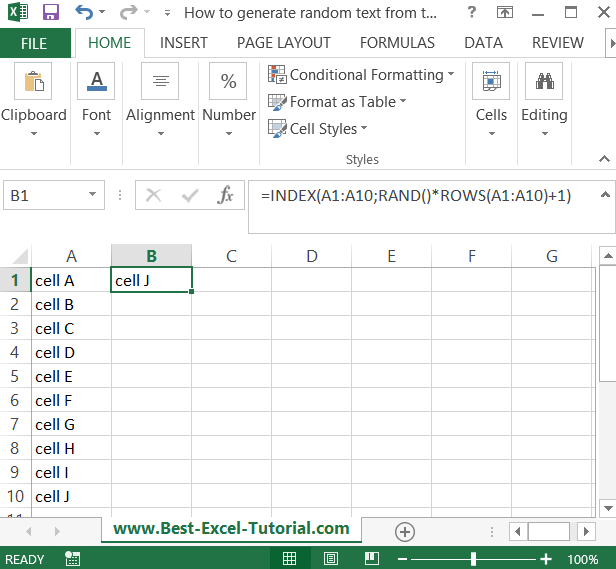#### How to generate random text from the list?

In this Excel tutorial, you will learn how to generate random text from a list.

## Random text generator formula

Suppose that your list is in the A1: A10 cell range. You have some text there from which you want to generate one in some other cell. Here is an Excel formula that solves your problem:

=INDEX(A1:A10,RAND()*ROWS(A1:A10)+1)Drag it down to the number of cells you need it in.

Remember that every time the formula generates a new text, Each time you run the formula, it will generate a random item from a given list.

## Random generator using ROUNDUP function

Another method would be to use ROUNDUP function.

1. Prepare your list: First, create a list of the text items you want to randomly generate. This list should be in a single column, with each item in a separate cell.
2. Enter the RAND function: In an adjacent column, enter the formula “=RAND()” in the first cell. This will generate a random number between 0 and 1.
3. Copy the formula: Copy the formula down the column, so that each cell in the column contains a unique random number.
4. Enter the INDEX function: In the next column, enter the formula “=INDEX(A1:A6,ROUNDUP(B1*6,0)),” replacing “A1:A6” with the range that contains your list of text items and “6” with the number of items in your list.
5. Copy the formula: Copy the formula down the column, so that each cell in the column contains a randomly selected text item from your list.
6. Finalize the random text: You can now use the randomly generated text in your Excel worksheet as needed. If you need to generate new random text, simply copy the “RAND” and “INDEX” formulas down the column again.
See also  How to Apply a Formula to an Entire Column in Excel

These steps should help you generate random text from a list in Excel. You can use this technique to randomly select items from any list, such as names, dates, or numbers, depending on the type of data you’re working with and the message you want to convey.

## How to Generate Random Text from a List Using the RANDBETWEEN Function

The RANDBETWEEN function is a built-in function in Excel that can be used to generate a random number between two values. The syntax of the RANDBETWEEN function is as follows:

`=RANDBETWEEN(start_value, end_value)`

For example, the following formula would generate a random number between 1 and 10:

`=RANDBETWEEN(1, 10)`

The RANDBETWEEN function can be used to generate random text from a list by using the INDEX function. The INDEX function returns the value from a range of cells based on a row and column number.

The following formula would generate a random text from the list in the range A1:A10:

`=INDEX(A1:A10,RANDBETWEEN(1,ROWS(A1:A10)))`

This formula first generates a random number between 1 and the number of rows in the range A1:A10. The INDEX function then returns the value from the list based on the random number.